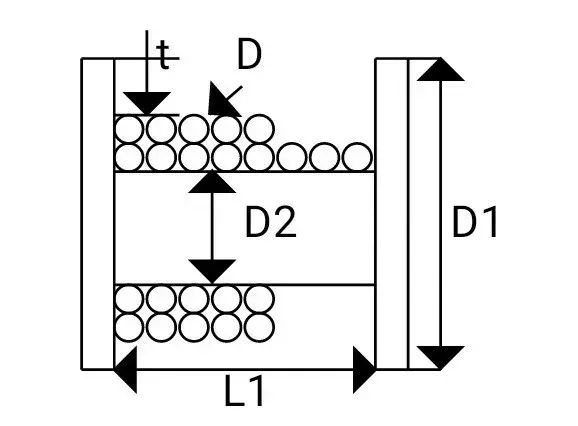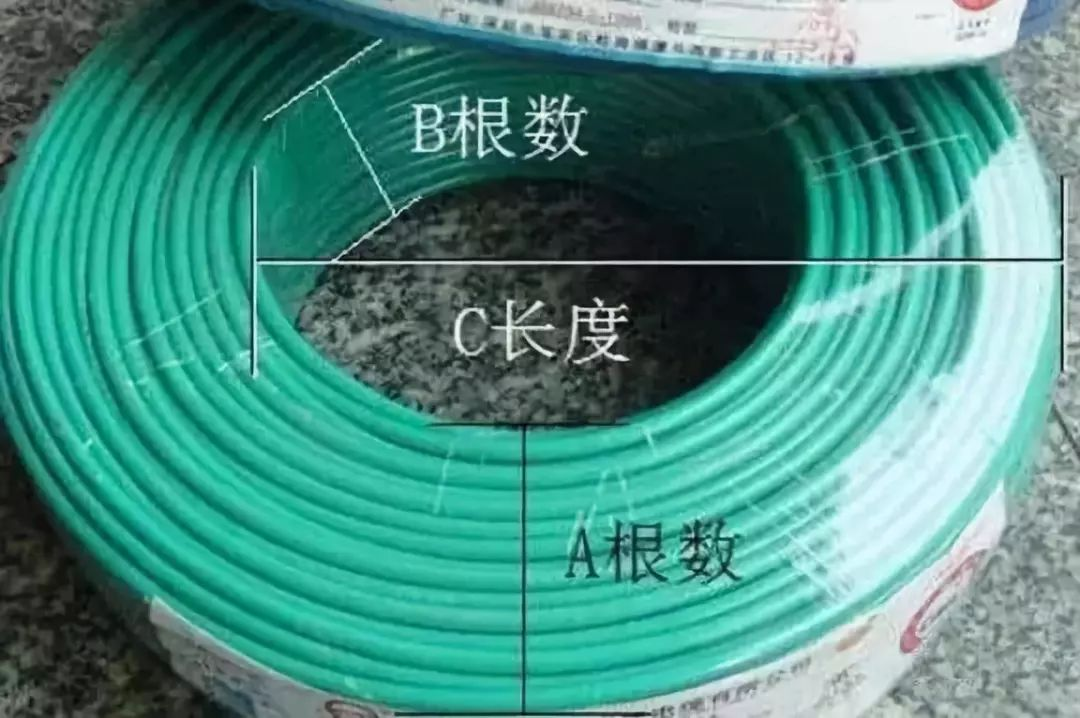### 首页 > 成缆 绕包 填充 > 一种新的电缆盘装线长度计算方法降包装成本

#### [工装盘具 ] 一种新的电缆盘装线长度计算方法降包装成本

##### zhuxuny##### P: 2019-03-30 09:29:13 1

1）电缆盘自身的稳定性，即包装盘在装电线电缆产品时，包装盘不变形，经得起吊卸；

2）电缆盘的肚径要满足被包装电缆产品的弯曲半径；

3）电缆盘要能装下被包装电缆特定长度的产品；

1π/4*D2*L=π/4*[(D1-2t)2-D22]

L=[(D1-2t)2- D22]/ D2・・・・・・・・・

L=0.87*[（D1-2t）2- D22]/ D2・・・・・・・・・

2

n=L1/D×0.95(系数0.95考虑实际情况排线不齐)・・・・・・・・・

P=(D1-D2-2*t)/(2*D)・・・・・・・・・

L’= p0π(D2+2D*x)dx=π(D2*x+D*2)P0

=π(D2*p+D*P2)・・・・・・・・・

L=n* L’=π*n*( D2*p+D*P2)/1000

=π*n*p*( D2+D*P)/1000・・・・・・・・・

3

L”=π[D2+(D1-D2-2*t)/2]

=π(D1+D2-2*t)/2・・・・・・・・・

n=L1/D×0.95・・・・・・・・・

(系数0.95考虑实际情况排线不齐，计算结果取整数部分值）

P=(D1-D2-2*t)/(2*D)・・・・・・・・・11

（计算结果取整数部分值)

L=n*p* L”

=π* n*p*(D1+D2-2*t)/2000・・・・・・・・・12

D2+D*P与(D1+D2-2*t)/2

=2*P*D+D2・・・・・・・・・13

B=(D2+2*P*D+D2)/2=(2*D2+2*P*D)/2

=D2+D*P=A

4

L”=π[D2+(D1-D2-2*t)/2]

= π(D1+D2-2*t)/2・・・・・・・・・14

n=L1/a×0.95・・・・・・・・・15

(系数0.95考虑实际情况排线不齐，计算结果取整数部分值)

P=(D1-D2-2*t)/(2* b)・・・・・・・・・16

（计算结果取整数部分值)

L=n*p* L”

=π* n*p*(D1+D2-2*t)/2000・・・・・・・・・17A根数：横面电线的根数（把电线横着放数出有多少根）

B根数：竖面电线的根数（把电线竖着放数出有多少根）

C长度：外围任意一边到内盘的长度（单位米）

#### single-fiber colouring unit - 单纤着色装置,单根光纤着色装置 点赞（0）投诉

##### xiao120#### convex polished ferrule endface - 抛光凸套管端面 点赞（0）投诉

##### wuziqi##### P: 2019-04-19 22:23:27 3#### shape-memory alloy wire - 形状记忆合金线 点赞（0）投诉

##### lxdgxl#### short dot - 短路点 点赞（0）投诉

##### 4